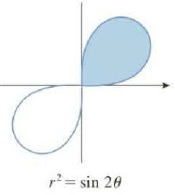Chapter 10.4, Problem 5E

Chapter
Section
Textbook Problem

# Find the area of the shaded region.5.To determine

To Find: The area of the shaded region shown in figure (1).

Explanation

Given:

The shaded region is shown as in the figure (1) below.

Calculation:

Write the formula for area as below.

A=ab12r2dθ=120π/2(sin2θ)dθ=120π/2(sin2θ)dθ=12[12

### Still sussing out bartleby?

Check out a sample textbook solution.

See a sample solution

#### The Solution to Your Study Problems

Bartleby provides explanations to thousands of textbook problems written by our experts, many with advanced degrees!

Get Started

#### In Exercises 7-12, solve for y in terms of x. x=2y13

Calculus: An Applied Approach (MindTap Course List)

#### Simplify the expression. 71. sin(tan1 x)

Single Variable Calculus: Early Transcendentals, Volume I

#### In Exercises 21-24, find the distance between the given points. 21. (1, 3) and (4, 7)

Applied Calculus for the Managerial, Life, and Social Sciences: A Brief Approach

#### Solve

Mathematical Applications for the Management, Life, and Social Sciences

#### Area In Exercises 73-76, find the area of the given region. y2=x2(1x2)

Calculus: Early Transcendental Functions (MindTap Course List)

#### Evaluate the indefinite integral. sec2xtan2xdx

Single Variable Calculus: Early Transcendentals